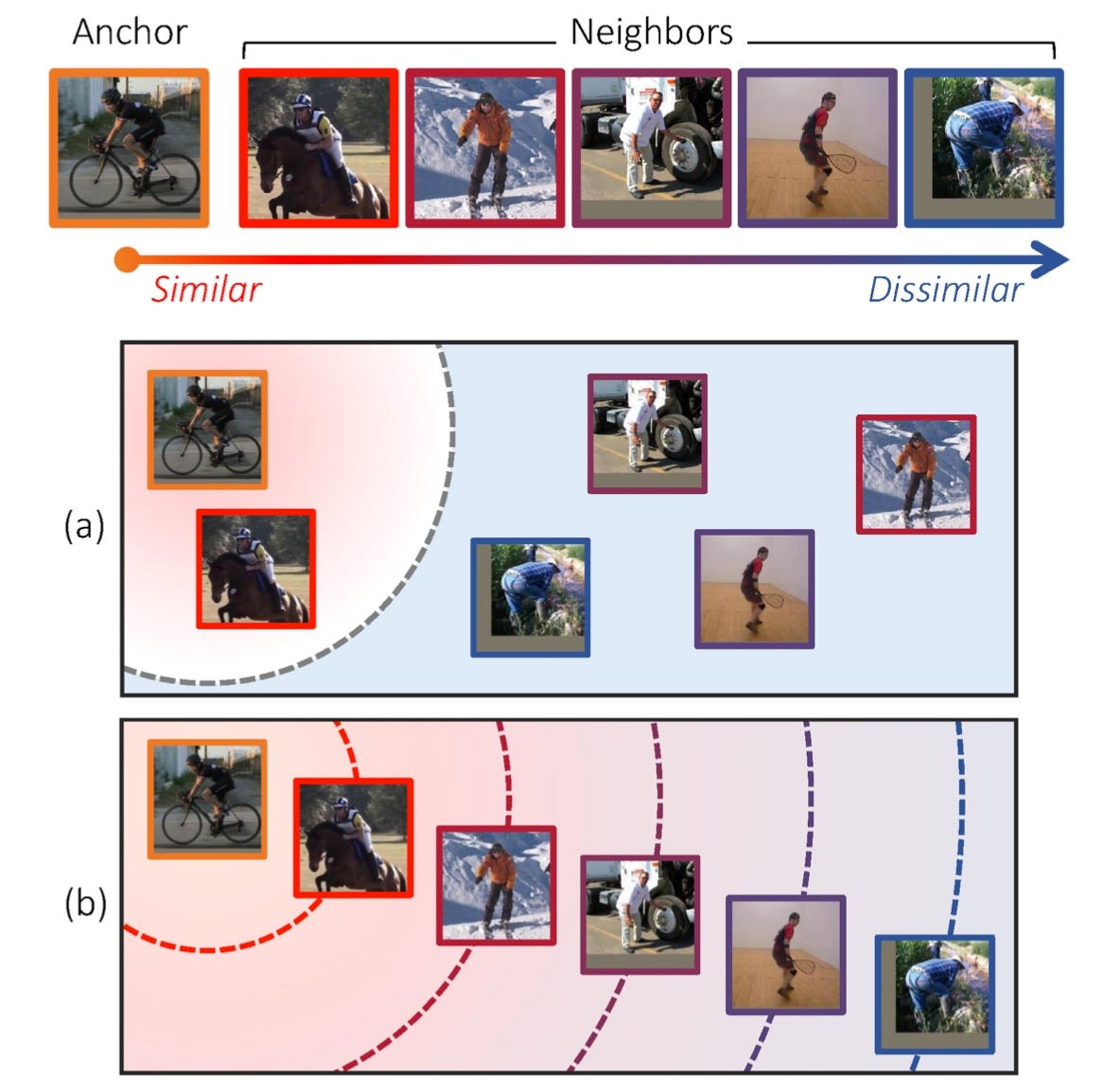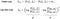Deep Metric Learning Beyond Binary Supervision

Metric learning literature assumes binary labels where samples belong to either the same or different classes. While this binary perspective has motivated fundamental ranking losses (e.g., Contrastive and Triplet loss), this binary perspective has reached a stagnant point . Thus, one novel direction for metric learning is continuous (non-binary) similarity. This paper  promotes metric learning beyond binary supervision as shown in the next Figure.(a) Existing methods categorize neighbors into positive and negative classes, and learn a metric space where positive images are close to the anchor and negative ones far apart. In such a space, the distance between a pair of images is not necessarily related to their semantic similarity since the order and degrees of similarities between them are disregarded. (b) This paper  allows distance ratios in the label space to be preserved in the learned metric space to overcome the aforementioned limitation.

The binary metric learning is not sufficient for objects with continuous similarity criteria such as image captions, human poses, and scene graphs. Thus, this paper  proposes a triplet loss variant, dubbed log-ratio loss, that takes full advantage of continuous labels. The log-ratio loss preserves ratios of similarity in the feature embedding space. The proposed loss is evaluated on three different image retrieval tasks: human poses, room layouts, and image captions.

The next Figure depicts both the vanilla triplet loss (TL) and the proposed log-ratio loss (LR).Triplet and Log-ratio losses. f indicates an embedding vector, y is a continuous label, D(·) denotes the squared Euclidean distance, m is a margin, and [·]+ denotes the hinge function. The embedding vectors are L2 normalized to avoid magnitudes’ divergence, i.e., the margin becomes trivial.

The log-ratio loss is similar to the standard regression loss (p`-p)² that minimizes the difference between the predicted p` and ground-truth p. If the distance between (y_a,y_i) is bigger than (y_a,y_j), then the distance between (f_a,f_i) should be bigger than (f_a,f_j), and vice versa. Please note the following two differences between triplet loss (TL) and log-ratio (LR): (1) TL operates on positive and negative samples (a, p, n), while LR creates triplets (a, i, j) without regarding the class labels. (2) TL requires a margin m, which is a hyperparameter tuned manually.

While log-ratio supports random mini-batch sampling, the paper proposes a dedicated mini-batch sampling strategy called dense-triplet-mining (DTM). DTM constructs a mini-batch B of training samples with an anchor, k nearest neighbors (kNNs) of the anchor in terms of label distance, and other neighbors randomly sampled from the remaining ones. The kNNs speed up training because a small D(y_a,y_i) induces large log-ratios of label distances. I elaborate more on DTM in the my-comments section at the end.

The following two figures present qualitative evaluations using human pose retrieval and caption-aware image retrieval.

The paper presents further quantitative evaluations to demonstrate the log-ratio superiority — these evaluations are omitted from this article. Yet, one interesting ablation study shows that log-ratio loss’s performance drops for small embedding dimensions marginally. The next figure shows how triplet loss (blue) suffers significantly with a small embedding dimension (d=16) while log-ratio remains resilient.

• The paper is well written and brings a novel perspective to the metric learning community.
• The authors released their code to Github.
• I am not sure why the authors use the log-ratio, and not just the ratio. Maybe because the feature embedding is L2-normalized. The maximum distance in an L2-normalized embedding is two, i.e., max(D(f_j,f_i))) = 2. Accordingly, the ratio between (y_i,y_j) needs normalization to a similar range.
• In metric learning, a large embedding dimension is vital for achieving superior performance. I am not aware of any paper that extensively studies the relationship between performance and embedding dimension: (1)why it happens? is it due to the L2 normalization? (2) how to mitigate it? Accordingly, the performance vs. embedding dimension evaluation (last figure) is interesting. I wish the authors had elaborated more on this.
• The authors supported log-ratio with a dedicated mini-batch mining strategy, dense-triplet-mining (DTM). The log-ratio loss supports random sampling. Thus, DTM is justified only if the random sampling is inferior. In Figure 3, the authors reported triplet loss performance with and without DTM. Yet, the log-ratio performance is never reported without DTM! Besides, DTM is not cheap. DTM loads the k nearest neighbors (kNNs) for every anchor. Finding these kNNs is not trivial for large datasets. An advanced data structure (e.g., Range Trees) is required to find these kNNs efficiently.

References

 Kim, Sungyeon, Minkyo Seo, Ivan Laptev, Minsu Cho, and Suha Kwak. “Deep metric learning beyond binary supervision.” CVPR 2019.

 Musgrave, Kevin, Serge Belongie, and Ser-Nam Lim. “A Metric Learning Reality Check.” ECCV 2020.

I write reviews on computer vision papers. Writing tips are welcomed.